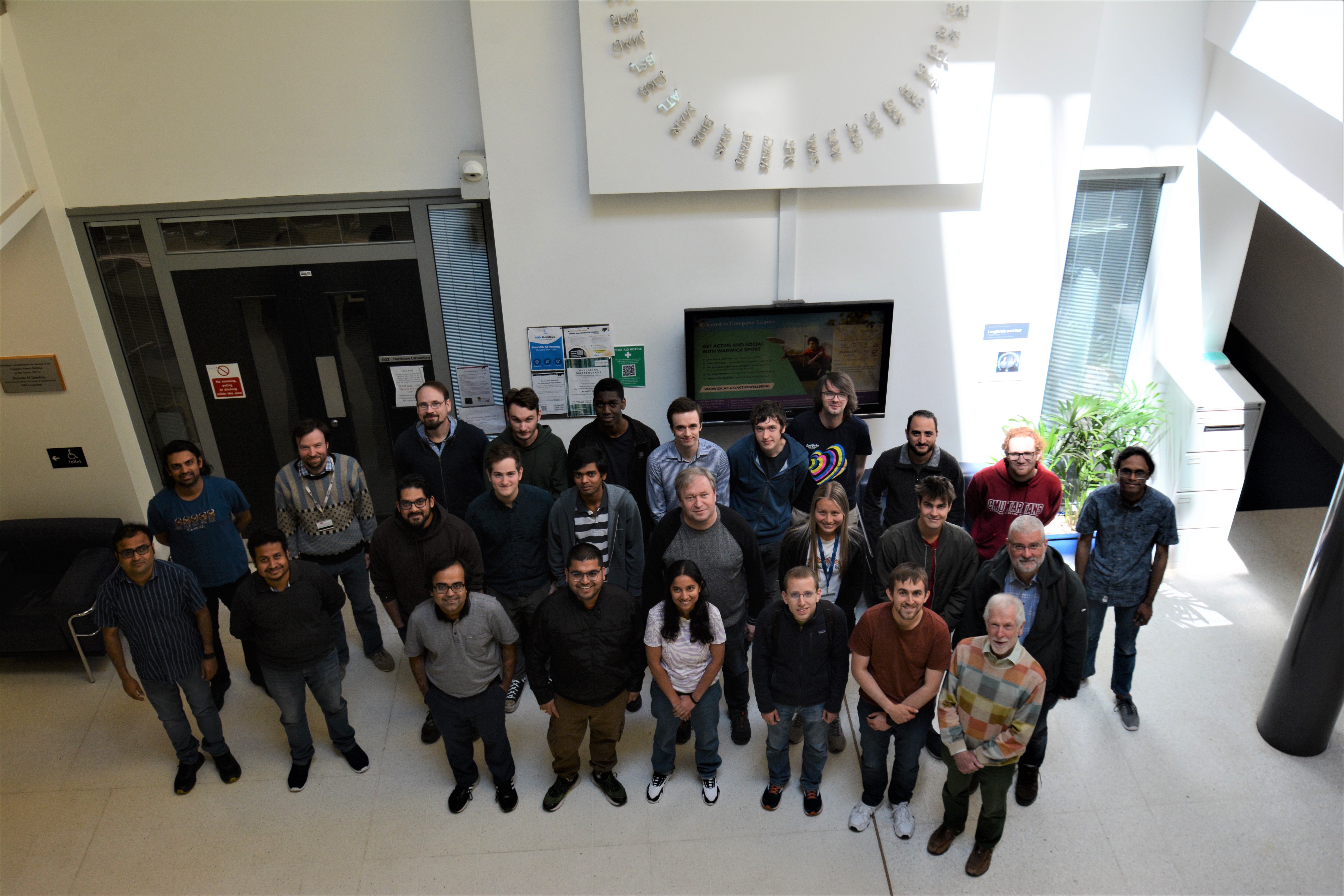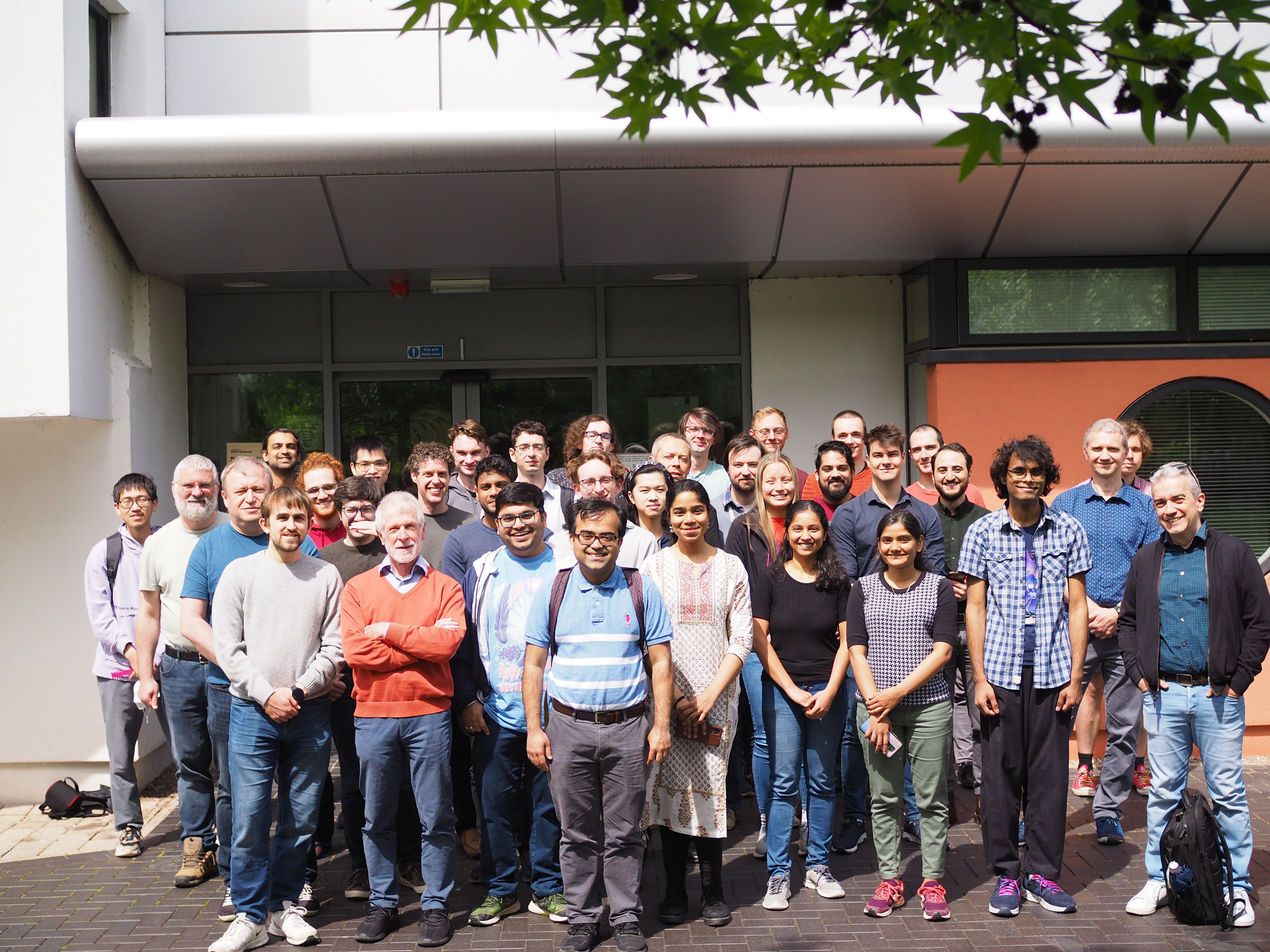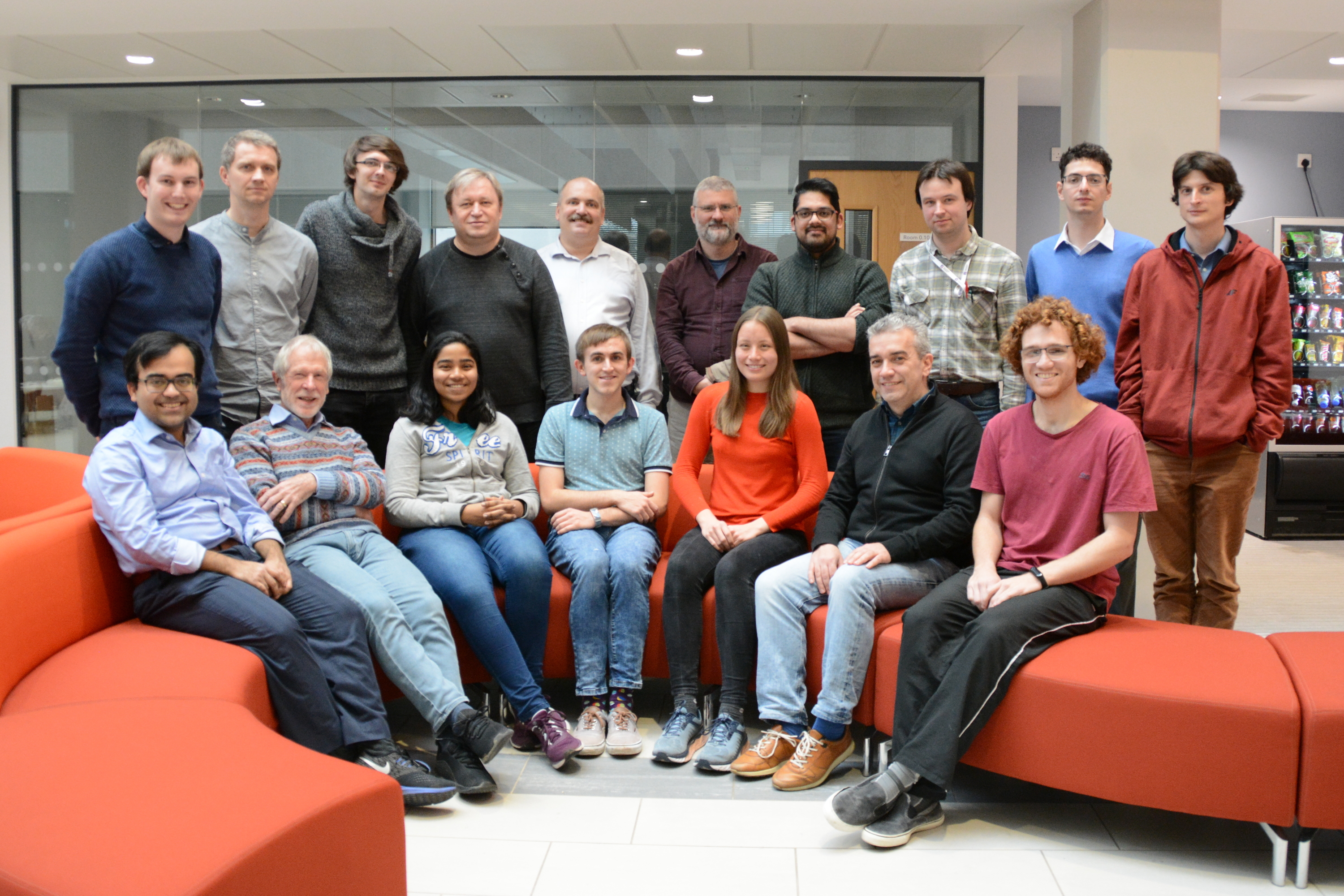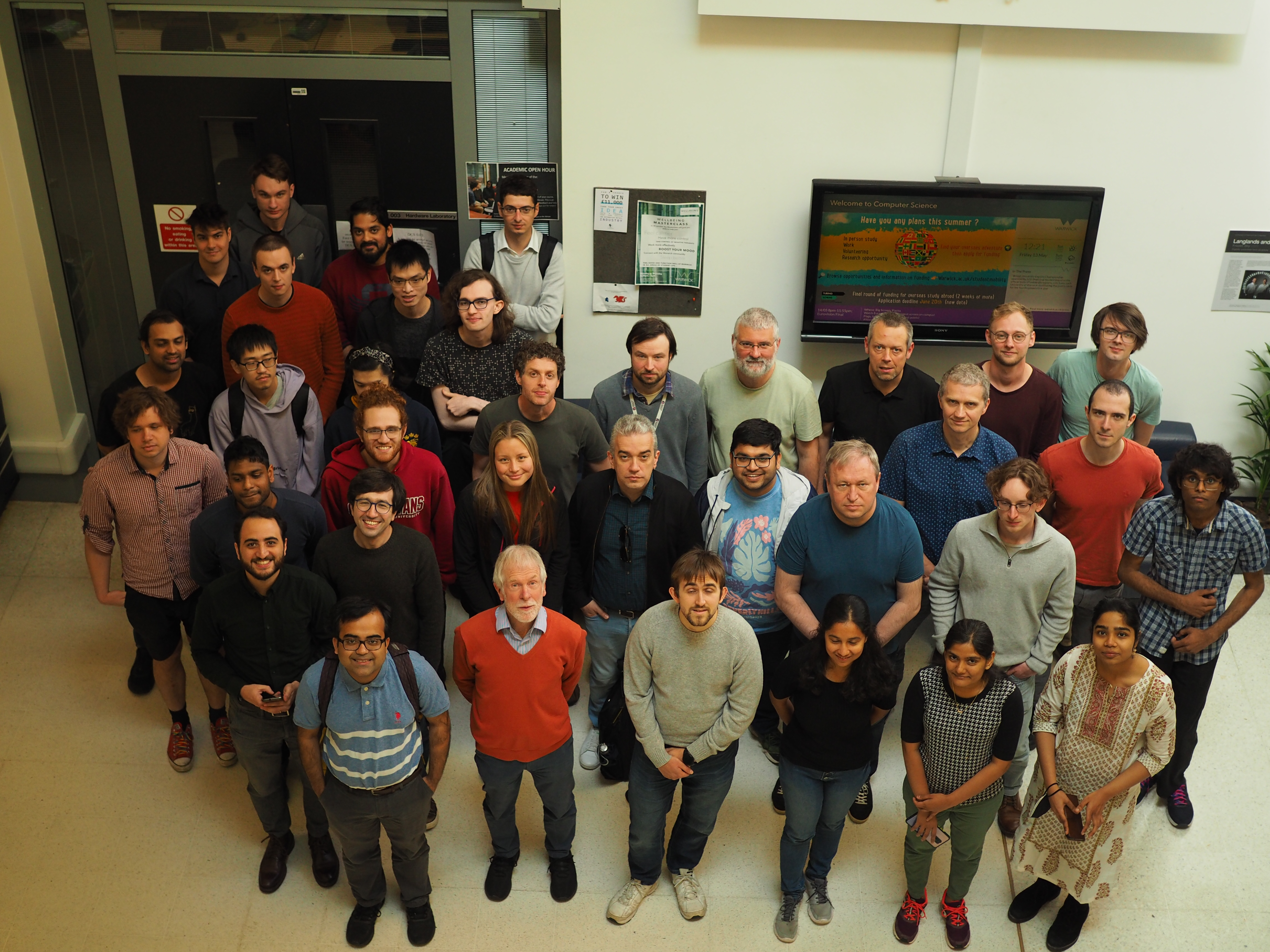# FoCS People

## Faculty

Sayan Bhattacharya Dynamic graph algorithms, data structures, online algorithms, streaming algorithms, algorithmic game theory
Dmitry Chistikov Automata and formal languages, verification, discrete mathematics
Graham Cormode Algorithms, databases, data streaming, big data, data science
Artur Czumaj (Head) Randomized and approximation algorithms, algorithmic game theory
Alex Dixon Automata theory, teaching the fundamentals of computer science
Charilaos Efthymiou Intersection of theory of algorithms, discrete mathematics, and statistical physics
Matthias Englert Online algorithms, approximation algorithms
Tom Gur Quantum computing, sublinear algorithms, complexity theory
Christian Ikenmeyer Computational complexity theory, tensor rank, computational representation theory, algebraic combinatorics, algebraic geometry
Marcin Jurdziński Algorithms, games, automata, logic
Dan Král' Graph theory, discrete mathematics, combinatorics
Ranko Lazic (Deputy) Theoretical computer science, machine learning
Torsten Mütze Graph theory, combinatorics, discrete algorithms, and their applications to real-world problems
Igor Carboni Oliveira Computational complexity theory and its connections to algorithms, combinatorics, and mathematical logic
Mike Paterson Combinatorial algorithms and complexity
Nick Spooner Interactive proof systems and zero knowledge in general, post-quantum cryptography, quantum information, coding theory and computational complexity
Ramanujan Sridharan Algorithms and complexity, fixed-parameter and approximation algorithms with a focus on graph and constraint satisfaction problems

## Research Fellows

Václav Blažej Algorithms
Nathanaël Fijalkow Complexity (algorithms), data structures, theory of programming languages, logic, algebra, databases, control theory
Siddharth Gupta Graph algorithms, computational geometry
Zhenjian Lu Computational complexity
Ian Mertz Computational complexity
Gopinath Mishra Algorithms
Anish Mukherjee Algorithms
Sathyawageeswar Subramanian Quantum algorithms and complexity theory## Research Students

Hugo Aaronson Quantum computing and complexity theory
Ari Biswas Differential privacy, probabilistic proof systems
Matthew Bowditch Machine learning
Andrei Codreanu Machine learning, theoretical computer science
Sam Coy Algorithms
Martin Costa Algorithms
Amelia Chui Algorithms
Marcel Dall’Angol Quantum computing and coding theory
Jacques Dark Streaming algorithms
Charlie Dickens Summaries and randomised algorithms for fundamental matrix computations and related problems
Chris Hickey Verification of data analysis algorithms
Peter Kiss Algorithms
Arturo Merino Algorithms and discrete mathematics
Namrata Algorithms, complexity and discrete mathematics
Jack O'Connor Quantum computing and learning theory
Bruno Pasqualotto Cavalar Computational complexity and combinatorics
Aditya Prakash Algorithms for automata and games
Mary Scott Differential privacy
Henry Sinclair-Banks Automata, complexity, and logic
Thejaswini K S Automata and algorithmic game theory
Peter Strulo Fixed-parameter algorithms
Dimitrios Tsintsilidas Computational complexity

Some new positions are available through the Centre for Discrete Mathematics and its Applications (DIMAP).